Tamilnadu State Board New Syllabus Samacheer Kalvi 12th Business Maths Guide Pdf Chapter 5 Numerical Methods Ex 5.1 Text Book Back Questions and Answers, Notes.

## Tamilnadu Samacheer Kalvi 12th Business Maths Solutions Chapter 5 Numerical Methods Ex 5.1

Question 1.
Evaluate Δ (log ax).
Solution:
Δ log (ax) = log (ax + h) – log ax
= log [ $$\frac { ax+h }{ax}$$ ] = log[$$\frac { ax }{ax}$$ + $$\frac { h }{ax}$$]
= log [1 + $$\frac { h }{ax}$$]Question 2.
If y = x³ – x² + x -1 calculate the values of y for x= 0, 1, 2, 3, 4, 5 and form the forward differences table.
Solution:
Given y = x³ – x² + x – 1
when x = 0 y = -1
when x = 1
y = 1 – 1 + 1 – 1 = 0
when x = 2
y = 8 – 4 + 2 – 1 = 5
for x = 0, 1, 2, 3, 4, 5
when x = 3
y = 27 – 9 + 3 – 1 = 20
when x = 4
y = 64 – 16 + 4 – 1 = 51
when x = 5
y = 125 – 25 + 5 – 1 = 104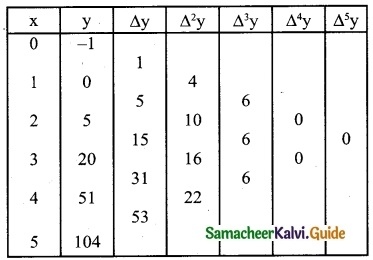Question 3.
If h = 1 then prove that (E-1 Δ)x³ = 3x² – 3x + 1
Solution:
h = 1 To prove (E-1 ∆) x3 = 3×2 – 3x + 1
L.H.S = (E-1 ∆) x3 = E-1 (∆x3)
= E-1[(x + h)3 – x3]
= E-1( x + h)3 – E-1(x3)
= (x – h + h)3 – (x – h)3
= x3 – (x – h)3
But given h = 1
So(E-1 ∆) x3 = x3 – (x – 1)3
= x3 – [x3 – 3x2 + 3x – 1]
= 3x2 – 3x + 1
= RHSQuestion 4.
If f(x) = x² + 3x than show that Δf(x) = 2x + 4
Solution:
Given f(x) = x³ + 3x; h = 1
Δf(x) = f (x + h) – f(x)
= (x + 1)² + 3 (x + 1) – (x² + 3x)
= x² + 2x + 1 + 3x + 3 – x² + 3x
= 2x + 4
∴ Δf(x) = 2x + 4

Question 5.
Evaluate Δ [ $$\frac { 1 }{(x+1)+(x+2)}$$ ] by taking ‘1’ as the interval of differencing
Solution: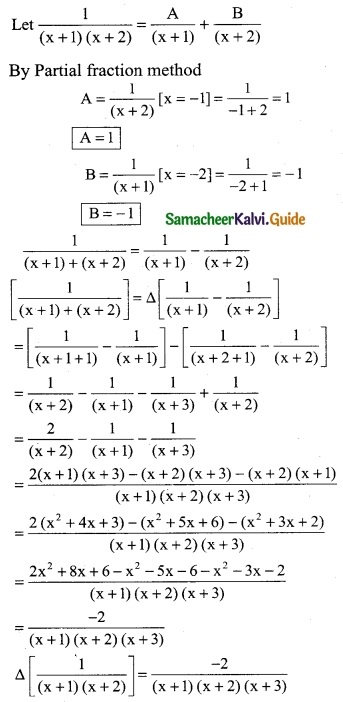Question 6.
Find the missing entry in the following table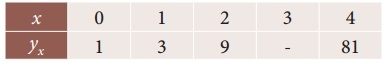Solution:
Since only four values of f(x) are given the polynomial which fits the data is of degree three. Hence fourth differences are zeros.
(ie) Δ4 y0 = 0
(E – 1)4 y0 = 0
(E4 – 4E³ + 6E² – 4E + 1) y0 = 0
E4y0 – 4E³ y0 + 6E²y0 – 4E y0 + 1y0 = 0
y4 – 4y3 + 6y2 – 4y1+ y0 = o
81 – 4y3 + 6(9) – 4(3) + 1 = 0
81 – 4y3 + 54 – 12 + 1 = 0
136 – 12 – 4y3 = 0
4y3 = 124
y3 = $$\frac { 124 }{4}$$
∴ y3 = 31Question 7.
Following are the population of a district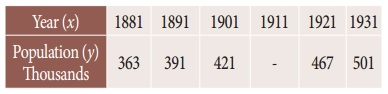Find the population of the year 1911
Solution:
Since only five values of fix) are given, the polynomial which fits the data is of degree four. Hence fifth differences are zeros.
(ie) Δ5 y0 = 0
(E – 1)5 y0 = 0
(E5 – 5E4 + 10E³ – 10E² + 5E – 1) y0 = 0
E5y0 – 5E4y0 + 10E³y0 – 10E²y0 + 5E y0 – y0 = 0
y5 – 5y4 + 10y3 – 10y2 + 5y1 – y0 = 0
501 – 5 (467) + 10(y3) -10 (421) + 5 (391) – 363 = 0
2456 – 6908 + 10y3 = 0
-4452 + 10y3 = 0 ⇒ 10y3 = 4452
y = $$\frac { 4452 }{10}$$ = 445.2
The population of the year 1911 is 445.2 thousandsQuestion 8.
Find the missing entries from the following.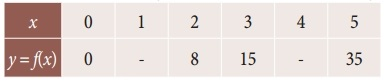Solution:
Since four values of f(x) are given we assume the polynomial of degree three. Hence fourth order differences are zeros.
(ie) Δ4y0 = 0 (ie) (E – 1)4 yk = 0
(E4 – 4E³ + 6E² – 4E + 1) yk = 0 ……… (1)
Put k = 0 in (1)
(E4 – 4E³ + 6E² – 4E + 1) y0 = 0
E4y0 – 4E³y0 + 6E³y0 – 4Ey0 + y0 = 0
y4 – 4y3 + 6y2 – 4y1 + y0 = 0
y4 – 4 (15) + 6(8) – 4y1 + 0 = 0
y4 – 4y1 = 12 …….. (2)
Put k = 1 in eqn (1)
(E4 – 4E³ + 6E² – 4E + 1) y1 = 0
y5 – 4y4 + 6y3 – 4y2 + y1 = 0
35 – 4 (y4) + 6(15) – 4(8) + y1 = 0
35 – 4y4 + 90 – 32 + y1 = 0
-4y4 + y1 + 125 – 32 = 0
-4y4 + y1 = -93 ………. (3)
Solving eqn (2) & (3)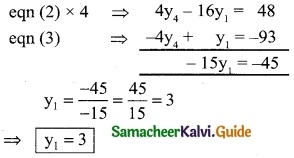Substitute y = 3 in eqn (2)
y4 – 4(3) = 12
y4 – 12 = 12
y4 = 12 + 12
∴ y4 = 24
The required two missing entries are 3 and 24.